Courses

# Critical Thickness of Insulation & Heat Transfer From Extended Surfaces (Fins) - MCQ Test 1

## 15 Questions MCQ Test RRB JE for Mechanical Engineering | Critical Thickness of Insulation & Heat Transfer From Extended Surfaces (Fins) - MCQ Test 1

Description
This mock test of Critical Thickness of Insulation & Heat Transfer From Extended Surfaces (Fins) - MCQ Test 1 for Mechanical Engineering helps you for every Mechanical Engineering entrance exam. This contains 15 Multiple Choice Questions for Mechanical Engineering Critical Thickness of Insulation & Heat Transfer From Extended Surfaces (Fins) - MCQ Test 1 (mcq) to study with solutions a complete question bank. The solved questions answers in this Critical Thickness of Insulation & Heat Transfer From Extended Surfaces (Fins) - MCQ Test 1 quiz give you a good mix of easy questions and tough questions. Mechanical Engineering students definitely take this Critical Thickness of Insulation & Heat Transfer From Extended Surfaces (Fins) - MCQ Test 1 exercise for a better result in the exam. You can find other Critical Thickness of Insulation & Heat Transfer From Extended Surfaces (Fins) - MCQ Test 1 extra questions, long questions & short questions for Mechanical Engineering on EduRev as well by searching above.
QUESTION: 1

### A steel steam pipe 10 cm inner diameter and 11 cm outer diameter is covered with insulation having the thermal conductivity of 1 W/mK. If the convective heat transfer coefficient between the surface of insulation and the surrounding air is 8 W / m2K, then critical radius of insulation is:

Solution:

Ans. (c) Critical radius of insulation (rc) =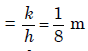= 12.5cm

QUESTION: 2

### For a current wire of 20 mm diameter exposed to air (h = 20 W/m2K),maximum heat dissipation occurs when thickness of insulation (k = 0.5W/mK) is:

Solution:

Ans. (b) Maximum heat dissipation occurs when thickness of insulation is critical.
Critical radius of insulation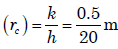= 25mm

Therefore thickness of insulation =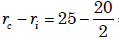= 15 mm

QUESTION: 3

### Upto the critical radius of insulation:

Solution:

Ans. (a)

QUESTION: 4

The value of thermal conductivity of thermal insulation applied to ahollow spherical vessel containing very hot material is 0·5 W/mK. The convective heat transfer coefficient at the outer surface of insulation is10 W/m2K.What is the critical radius of the sphere?

Solution:

Ans. (a) Minimum q at ro =
(k/h) = rcr (critical radius)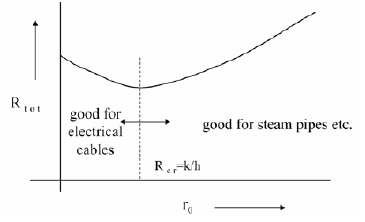QUESTION: 5

A metal rod of 2 cm diameter has a conductivity of 40W/mK, which is tobe insulated with an insulating material of conductivity of 0.1 W/m K. Ifthe convective heat transfer coefficient with the ambient atmosphere is5 W/m2K, the critical thickness of insulation will be:

Solution:

Ans. (a) Critical radius of insulation (rc) =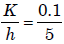= 0.02m = 2cm

Critical thickness of insulation (t) =rc - r1 = 2 - 1 = 1cm

QUESTION: 6

In order to substantially reduce leakage of heat from atmosphere intocold refrigerant flowing in small diameter copper tubes in a refrigerant system, the radial thickness of insulation, cylindrically wrapped around the tubes, must be:

Solution:

Ans. (d) At critical radius of insulation heat leakage is maximum if we add more
insulation then heat leakage will reduce.

QUESTION: 7

In a long cylindrical rod of radius R and a surface heat flux of qo the uniform internal heat generation rate is:

Solution:

Ans. (a)

QUESTION: 8

A plane wall of thickness 2L has a uniform volumetric heat source q* (W/m3). It is exposed to local ambient temperature T at both the ends (x = ± L). The surface temperature Ts of the wall under steady-state
condition (where h and k have their usual meanings) is given by:

Solution:

Ans. (a)

QUESTION: 9

A steam pipe is to be lined with two layers of insulating materials of different thermal conductivities. For minimum heat transfer

Solution:

Ans. (a) For minimum heat transfer, the better insulation must be put inside.

QUESTION: 10

An electric cable of aluminium conductor (k = 240 W/mK) is to be insulated with rubber (k = 0.15 W/mK). The cable is to be located in air (h = 6 W/m2). The critical thickness of insulation will be

Solution:
QUESTION: 11

Assertion (A): Addition of insulation to the inside surface of a pipealways reduces heat transfer rate and critical radius concept has nosignificance.
Reason (R): If insulation is added to the inside surface, both surfaceresistance and internal resistance increase.

Solution:

Ans. (a) A and R are correct. R is right reason for A.

QUESTION: 12

It is desired to increase the heat dissipation rate over the surface of anelectronic device of spherical shape of 5 mm radius exposed toconvection with h = 10 W/m2K by encasing it in a spherical sheath ofconductivity 0.04 W/mK, For maximum heat flow, the diameter of thesheath should be:

Solution:

Ans. (b) The critical radius of insulation for ensuring maximum heat transfer by
conduction (r) =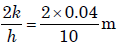= 8mm.

Therefore diameter should be 16 mm.

QUESTION: 13

From a metallic wall at 100°C, a metallic rod protrudes to the ambientair. The temperatures at the tip will be minimum when the rod is made of:

Solution:

Ans. (b)

QUESTION: 14

Usually fins are provided to increase the rate of heat transfer. But finsalso act as insulation. Which one of the following non-dimensional numbers decides this factor?

Solution:
QUESTION: 15

Which one of the following is correct?
Fins are used to increase the heat transfer from a surface by

Solution:

Ans. (b)